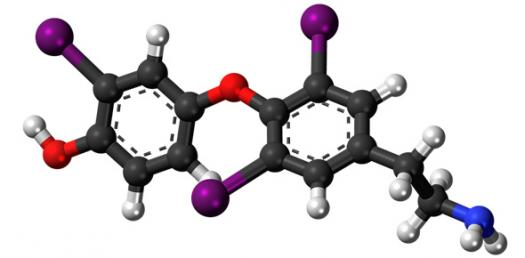# Quiz Over Atoms And Molecules

15 Questions | Total Attempts: 1644SettingsAtoms are the smallest particle into which an element can be divided. Atoms can join together to form molecules, which in turn form most of the objects around you. The quiz below should take you less than thirty minutes and will help you understand the two particles better. Try it out!

• 1.
Which of the following correctly represents 360g of water?a) 2 moles of waterb) 20 moles of waterc) 6.022 x 1023 molecules of waterd) 1.2044 x 1025 molecules of water
• A.

A)

• B.

A) and d)

• C.

B) and c)

• D.

B) and d)

• 2.
Which one of the following statement is not true about an atom​
• A.

Atoms are not able to exist independently

• B.

Atoms are the basic units from which molecules and ions are formed

• C.

Atoms are always neutral

• D.

Atoms aggregate in large numbers to form the matters that we can see, feel or touch

• 3.
What is the ratio of hydrogen and oxygen in water
• A.

1: 8

• B.

2:12

• C.

2:3

• D.

1:2

• 4.
An example  of polyatomic molecule
• A.

Nitrogen

• B.

Hydrogen

• C.

Sulphur

• D.

Oxygen

• 5.
All samples of carbon dioxide contain carbon and oxygen in the mass ratio of 3:8. This is in agreement with the Law of ___________.
• A.

Conservation of Mass

• B.

Constant Proportion

• C.

Multiple Proportion

• D.

Reciprocal Proportion

• 6.
The smallest particle of a substance that is capable of independent existence is _________.
• A.

Atom

• B.

Molecule

• C.

Electron

• D.

Proton

• 7.
Avogadro number represents the number of atoms in ________.​
• A.

12 grams of 12C

• B.

320 grams of sulphur

• C.

32 grams of oxygen

• D.

1 gram of 12C

• 8.
A sample contains 22 g of carbon dioxide. This is equal to​
• A.

One molar volume of carbon dioxide

• B.

One mole of carbon dioxide

• C.

Half mole of carbon dioxide

• D.

Two moles of carbon dioxide

• 9.
Select the ionic compound from the following
• A.

Sulphur molecule,

• B.

Phosphorous molecule,

• C.

Methane,

• D.

Copper nitrate,

• 10.
Chose the option which does not change when a chemical reaction takes place
• A.

Volume

• B.

Mass

• C.

Physical properties

• D.

Chemical properties

• 11.
Select the option which does not represent molar mass of a substance?
• A.

1 mole of HCl

• B.

6.022 x molecules of helium

• C.

16 gm of

• D.

44 gm of

• 12.
Select the case where formula mass is not used​
• A.

NaCl

• B.
• C.
• D.

CaO

• 13.
The average atomic mass of a sample of an element X is 16.2 u. What is the % of isotopes  8X16   and  8X18 in the sample?​
• A.

20%

• B.

10%

• C.

50%

• D.

15%

• 14.
The chemical symbol for nitrogen gas is​
• A.

Ni

• B.
• C.

N+

• D.

N

• 15.
Which one of the following would weigh the highest?
• A.

0.2 mole of sucrose( )

• B.

2 moles of

• C.

2 moles of

• D.

10 moles of

Related TopicsBack to top